Select Page

# 12 Science Maths CBSE Answers for MCQ Vector Algebra in English

12 Science Maths CBSE Answers for MCQ Vector Algebra in English to enable students to get Answers in a narrative video format for the specific question.

Expert Teacher provides 12 Science Maths CBSE Answers for MCQ Vector Algebra through Video Answers in English language. This video solution will be useful for students to understand how to write an answer in exam in order to score more marks. This teacher uses a narrative style for a question from Vector Algebra not only to explain the proper method of answering question, but deriving right answer too.

Please find the question below and view the Answer in a narrative video format.

Question:

Answer Video in English:

You can select video Answers from other languages also. Please check Answers in ( Hindi )

## Similar Questions from CBSE, 12th Science, Maths, Vector Algebra

Question 1 : Find the scalar components of the vector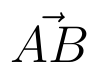with initial point A (2, 1) and terminal point B (-5, 7).  (View Answer Video)

Question 2 : Find the unit vector in the direction of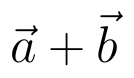if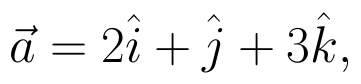and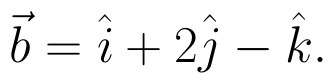(View Answer Video)

Question 3 : Find a unit vector parallel to the sum of vectors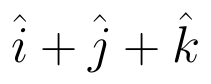and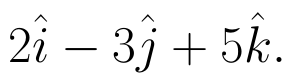(View Answer Video)

Question 4 : Write the position vector of the point which divides the join of points with position vectors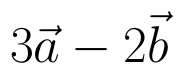and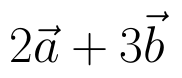in the ratio 2:1.  (View Answer Video)

Question 5 : Find the area of the triangle with vertices A(1, 1, 2), B(2, 3, 5) and C(1, 5, 5). (View Answer Video)

### Inverse Trigonometric Functions

Question 1 : Solve for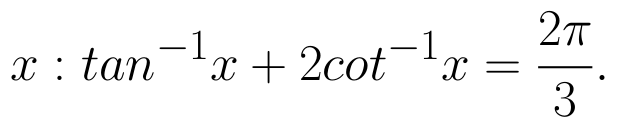(View Answer Video)

Question 2 : Evaluate :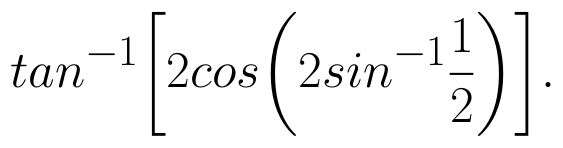(View Answer Video)

Question 3 : Write the value of the following: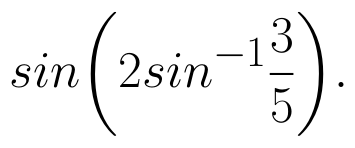(View Answer Video)

Question 4 : Evaluate :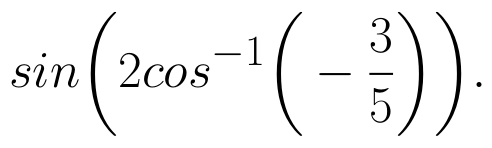(View Answer Video)

Question 5 : Write the value of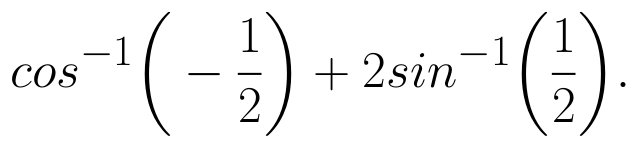(View Answer Video)

### Continuity and Differentiability

Question 1 :  Find the second order derivative of the function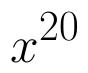. (View Answer Video)

Question 2 : Differentiate the function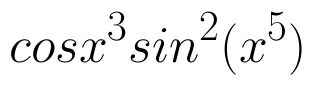with respect to x. (View Answer Video)

Question 3 : Differentiate the function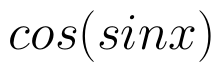with respect to x. (View Answer Video)

Question 4 : If x and y are connected parametrically by the equation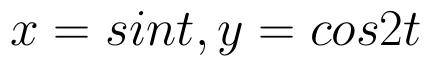, without eliminating the parameter, find. (View Answer Video)

Question 5 : Findfor the function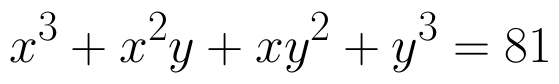. (View Answer Video)

### Determinant

Question 1 : Which of the following is correct? (View Answer Video)

Question 2 : Evaluate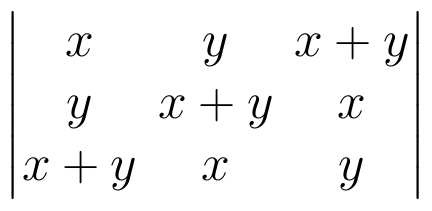. (View Answer Video)

Question 3 : If A =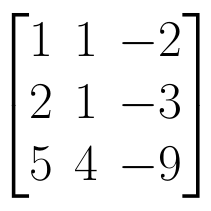, find | A |. (View Answer Video)

Question 4 :  Using the properties of determinants, evaluate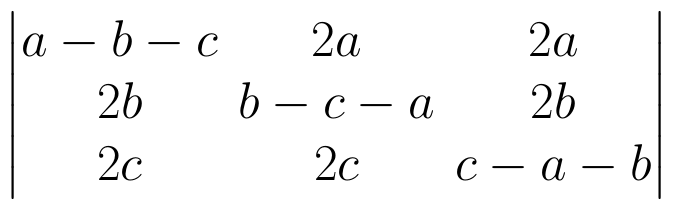. (View Answer Video)

Question 5 : If A is an invertible matrix of order 2, then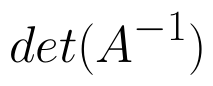is equal to, (View Answer Video)